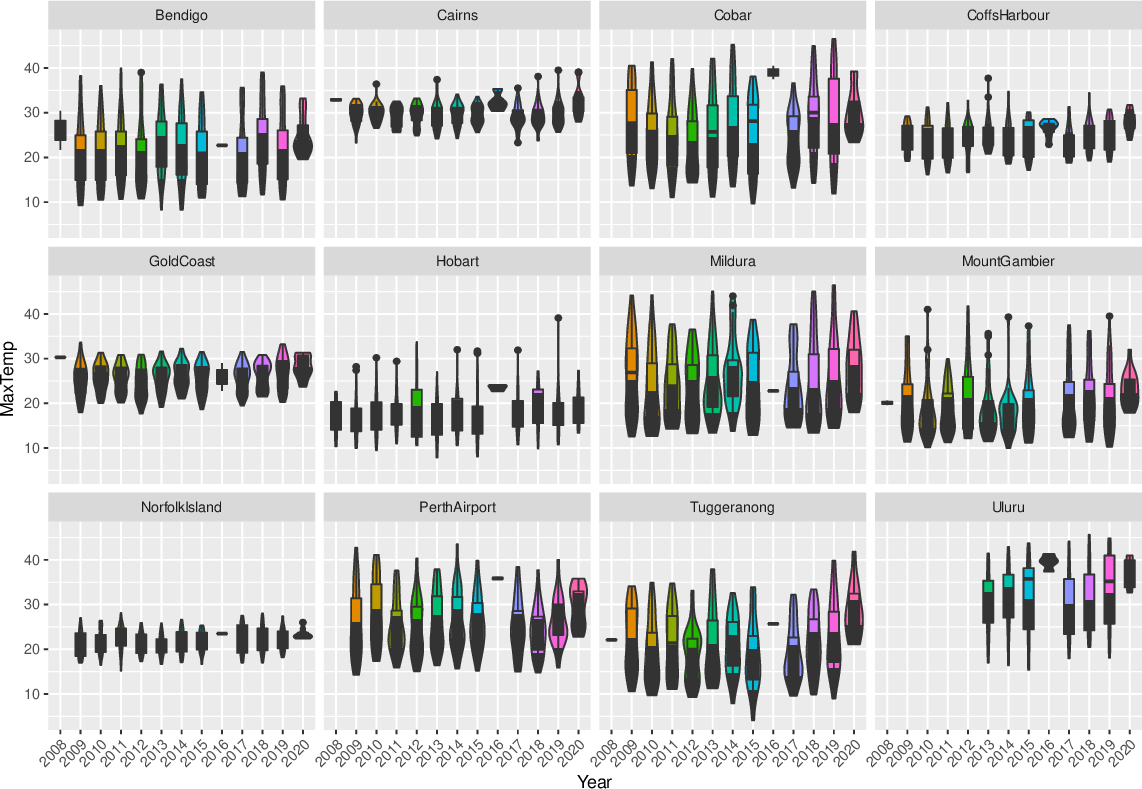Data Science Desktop Survival Guide by Graham WilliamsDesktop Survival Project Home Preface Data Science Introducing R R Constructs R Tasks R Strings R Read, Write, and Create Data Template Data Exploration Data Wrangling Data Visualisation Statistics ML Template ML Scenarios ML Activities ML Applications ML Algorithms Cluster Analysis Decision Trees Computer Vision Graph Data Privacy Literate Data Science Coding with Style Resources Bibliography Index

## Violin Plot Faceted Location

Rawds %>%   mutate(year=factor(format(ds\$date, "%Y"))) %>%   filter(location %in% (ds\$location %>% unique %>% sample(12))) %>%   sample_frac(0.1) %>%   ggplot(aes(x=year, y=max_temp, fill=year)) +   geom_violin() +   geom_boxplot(width=.5, position=position_dodge(width=0)) +   theme(legend.position="none") +   theme(axis.text.x=element_text(angle=45, hjust=1)) +   labs(x="Year", y=vnames["max_temp"]) +   facet_wrap(~location) We can readily split the plot across the locations. Things get a little crowded, but we get an overall view across all of the different weather stations. Notice we also rotated the x-axis labels so that they don't overlap. We can immediately see one of the issues with this dataset, noting that three weather stations have fewer observations that then others. Various other observations are also interesting. Some locations have little variation in their maximum temperatures over the years.